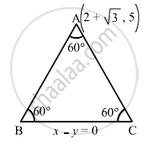Department of Pre-University Education, KarnatakaPUC Karnataka Science Class 11
Advertisement Remove all ads

# The Equation of One Side of an Equilateral Triangle is X − Y = 0 and One Vertex is ( 2 + √ 3 , 5 ) . Prove that a Second Side is Y + ( 2 − √ 3 ) X = 6 and Find the Equation of the Third Side. - Mathematics

Answer in Brief

The equation of one side of an equilateral triangle is x − y = 0 and one vertex is $(2 + \sqrt{3}, 5)$. Prove that a second side is $y + (2 - \sqrt{3}) x = 6$  and find the equation of the third side.

Advertisement Remove all ads

#### Solution

Let

$A\left( 2 + \sqrt{3}, 5 \right)$ be the vertex of the equilateral triangle ABC and x − y = 0 be the equation of BC.Here, we have to find the equations of sides AB and AC, each of which makes an angle of

${60}^\circ$ with the line x − y = 0

We know the equations of two lines passing through a point $\left( x_1 , y_1 \right)$ and making an angle $\alpha$ with the line whose slope is m.

$y - y_1 = \frac{m \pm \tan\alpha}{1 \mp m\tan\alpha}\left( x - x_1 \right)$

Here,

$x_1 = 2 + \sqrt{3}, y_1 = 5, \alpha = {60}^\circ , m = 1$

So, the equations of the required sides are

$y - 5 = \frac{1 + \tan {60}^\circ}{1 - \tan {60}^\circ}\left( x - 2 - \sqrt{3} \right) \text { and }y - 5 = \frac{1 - \tan {60}^\circ}{1 + \tan {60}^\circ}\left( x - 2 - \sqrt{3} \right)$

$\Rightarrow y - 5 = - \left( 2 + \sqrt{3} \right)\left( x - 2 - \sqrt{3} \right) \text { and } y - 5 = - \left( 2 - \sqrt{3} \right)\left( x - 2 - \sqrt{3} \right)$

$\Rightarrow y - 5 = - \left( 2 + \sqrt{3} \right)x + \left( 2 + \sqrt{3} \right)^2 \text { and } y - 5 = - \left( 2 - \sqrt{3} \right)x + \left( 2 - \sqrt{3} \right)\left( 2 + \sqrt{3} \right)$

$\Rightarrow \left( 2 + \sqrt{3} \right)x + y = 2 + 4\sqrt{3} \text { and } \left( 2 - \sqrt{3} \right)x + y - 6 = 0$

Hence, the second side is $y + (2 - \sqrt{3}) x = 6$ and the equation of the third side is $\left( 2 + \sqrt{3} \right)x + y = 12 + 4\sqrt{3}$
Concept: Straight Lines - Equation of Family of Lines Passing Through the Point of Intersection of Two Lines
Is there an error in this question or solution?
Advertisement Remove all ads

#### APPEARS IN

RD Sharma Class 11 Mathematics Textbook
Chapter 23 The straight lines
Exercise 23.18 | Q 7 | Page 125
Advertisement Remove all ads
Advertisement Remove all ads
Share
Notifications

View all notifications

Forgot password?